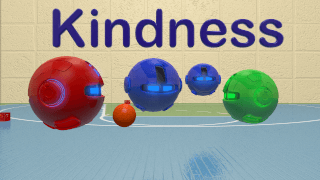Select Page

All our math videos are free to watch. With any of our paid memberships you also get access to worksheets and our videos go right along with the questions. You also get access to new interactive questions and any new items we continually add. Check out our paid memberships today!

In the video we teach about fractions and review basic fractions with numerators and denominators. We then jump into building simple equations to show how fractions are parts of a whole.

We learn to compare two different fractions for 4th Grade in this fun video. We use different methods to compare fractions with different denominators. Finding the LCD or least common denominator will help us solve these problems, and with a little magic too!

#### Fraction Word Problems

Come solve Fraction Word Problems with us and use our worksheets to test your comprehension. Solving word problems with fractions does not need to be scary so join us!

We learn to carry in this Multi-Digit Addition video for 4th grade kids. This math video helps kids use the standard algorithm to solve large addition problems. Carry the boxes carefully or they will spill the balls!

#### Multi Digit Subtraction

We learn about borrowing from the next place value in this fun video for kids in the 4th grade. Subtracting multi digit numbers can be made easier when a fun visual is presented, feel free to pause the video to ask questions and have kids follow along.

Let’s Add Mixed Numbers also called Mixed Fractions. It is cold outside in mixed number village so come warm up with as and learn how to add when you have a number and a fraction.

#### Subtracting Mixed Numbers

Come learn about Subtracting Mixed Numbers also called Mixed Fractions. Help us charge the Math Wasp as we learn how to subtract when we have a mixed number like 4 and 1/5.

#### Ten Times Place Value

Kids will learn about place value for 4th Grade. Our video teaches kids that each digit to the left of each other is ten times larger than the digit to the right. We hope you enjoy our math videos.

#### Multi Step Word Problems

Kids in 4th grade learn in this video how to solve multi step word problems. Many word problems and problems in life require more than one step. This video helps kids figure out the basics of multi step word problems. We hope you enjoy the video!

#### Multiply 4 Digits By One Digit

This video teaches kids how to multiply 4 digits by one digit. Large number multiplication is essential in 4th grade math. Remind the kids that practice makes Awesome in math!

#### Multiply 2 Digits By 2 Digits

This video teaches kids how to multiply 2 digits by two digits. Large Number multiplication is essential in 4th grade math. Remind the kids that practice makes Awesome in math!

#### Multiplicative Comparison

Kids will learn in this video to interpret a multiplication equation as a comparison. An example is 35 = 5 x 7 which means that 35 is 5 times as many as 7 and 7 times as many as 5.  Please use this video to assist your kids both in the classroom and at home.

#### Multiplying Fractions Word Problems

Kids will learn about multiplying fractions in word problems. We use visual fraction models and equations to represent the problems. Pause the video at any time to ask the kids questions.

#### Factor Pairs

Find all factor pairs for a while number in the range 1-100. We learn in this video that a whole number is a multiple of each of its factors. Enjoy the video! We will be making our own versions of all these videos soon.

#### Compare Decimal Numbers

Learn with us as we compare decimals to see which is greater than, less than and equal to. Learning the difference between decimals is a fun thing to do when using our game and video.

Come learn about Long Division for 4th Grade in this fun Math Video. We work through the basics of what many consider the traditional form of long division. Please pause the video at any time to help kids understand as it moves kind of quickly.

#### Long Division with Remainders

Kids will learn about Long Division with Remainders in this funny video involving the Number One who ate too much to avoid remainders. Kids will laugh and enjoy learning about remainders in long division.

### Here are some fun videos found in other grades that you might like to use in class.#### Controlling Emotions

Watch this video in class to help kids know how to control emotions.#### Kindness Video for Kids

This is a video we created to help kids learn kindness and self worth. It was designed for our youtube channel but we post all our videos here without ads. We hope kids learn that we are all different and that is what makes us amazing!